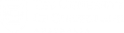# Applied Statistics for Water and Wastewater

The aim of this 18hr course is to teach fundamentals of inferential statistics with applied problems in water, wastewater, environmental monitoring, process monitoring, and public health.

The focus is on clearly presenting and translating statistic theory to practical case studies. Real data is analysed to make evidence based interpretation and conclusions for practical problems.

The course focuses on use of widespread tools (e.g., Microsoft excel), which enables the use of powerful statistical techniques.

Each session focuses on a core concept, generally integrating multi-mode teaching activities within the session, including shorter lectures, application of computing to analyse data, and team workshops.

### Fundamentals

• Fundamentals of inferential statistics – samples and populations. Measurement uncertainty vs variability. Introducing key measures in water and wastewater. Visualising data effectively.
• Use of distributions to analyse data and represent variability in population, and uncertainty in measurements. Concept of hypothesis testing to make evidence based conclusions. Limitations of inferential statistics and alternatives.
• Cause-effect analysis, and basic concepts of experimental design. Introduction of analysis of variance (ANOVA) to analyse cause-effect issues.
• Workshop on multiple case studies. Determine approach and make an evidence based analysis of typical wastewater and environmental monitoring problems.

### Applied statistics

• Cause-effect applied to input-output relationships. Use of linear regression in a wastewater context to assess relationships. Controlling for factors by mixing ANOVA and regression. Determining and presenting uncertainty in regression parameters and strength of relationships.
• Principles and applications of non-linear regression. Determining principal parameters. Case studies in both laboratory and field context (e.g., measuring Oxygen Uptake Rate, BOD etc).
• Uncertainty propagation principles. How to determine uncertainty in a calculated value. How does uncertainty in an input or parameter determine uncertainty in an output.
• Case study workshop. Using continuous applied statistics to analyse a key problem in the wastewater process.

### On the job

• Laboratory statistics. How can you trust your lab? What should you do in the lab? Using statistics in method development, QC, limit of detection, limit of measurement, validation, presenting data effectively.
• Experimental statistics (lab, field, and process). Determining control-experiment setups effectively to determine if a change has an effect. Field testing equipment effectively. Time-series vs parallel system evaluation.
• Process statistics. Big data visualisation and processing. Introduction to large data analytical techniques. Looking for relationships beyond the noise.
• Wrap-up. A couple of big picture examples.